Mathematical and Physical Journal
for High Schools
Issued by the MATFUND Foundation
 Already signed up? New to KöMaL?

# KöMaL Problems in Physics, December 2008

Show/hide problems of signs:## Problems with sign 'M'

Deadline expired on January 12, 2009.

M. 292. Determine experimentally how the retarding force exerted on a balloon falling in air depends on the speed of the balloon.

(6 pont)

statistics## Problems with sign 'P'

Deadline expired on January 12, 2009.

P. 4114. In which ball game is the ratio of the number of shots which hit the goal posts or the crossbar to the number of all shots is greater: in water polo or in handball? Why? (In both ball games the goal posts are at the same distances, and the goalposts and crossbars in both games have approximately the same thickness.)

(3 pont)

statistics

P. 4115. The third meter shown in the figure reads 6 V. What do the other meters measure and what are their readings? What is the voltage across the power supply? (The meters can be considered ideal ones.)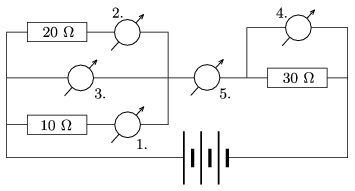(3 pont)

statistics

P. 4116. Prove that the following statement of Galilei (Galilei: Discourses and Mathematical Demonstrations Relating to Two New Sciences; Leyden (1938).) is true: The ratio of the elapsed time intervals while a point-like object slides without friction along the same lengths of slopes but of different angles of elevation is the same as the ratio of the reciprocals of the square roots of the corresponding heights of the slope.''

(3 pont)

statistics

P. 4117. In one of the pans of a two-pan balance there is a beaker containing some water and in the other pan there is a stand onto which an aluminium cylinder of mass 108 g is hanged. The balance is in equilibrium. If the thread is extended such that the aluminium cylinder is totally immersed into the water, the equilibrium is ceased. Find the mass of the weight which is to be put into the pan at the right in order to balance the scale.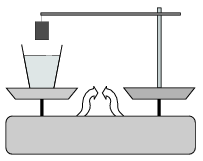(4 pont)

statistics

P. 4118. The plank of mass 2m shown in the figure can move without friction along a horizontal surface. The length of the thread of the pendulum fixed to the plank is L=20 cm, and the mass of the (point-like) bob at the end of the thread is m. The bob is displaced such that the angle between the thread and the vertical is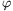=60o, and then it is released.

a) Find the velocity of the bob when the thread is vertical.

b) Find the velocity of the plank when the angle between the thread and the vertical is 45o.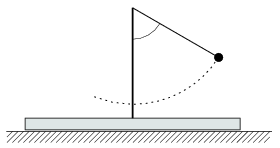(5 pont)

statistics

P. 4119. The weighing scale with which the mass of animals can be measured is a cage which has pressure sensors below its four vertices on its bottom. These sensors continuously transmit the measured data to a computer. From the measured data can the computer calculate the mass of the moving animal in the cage? Is it possible to find the mass if the animal is leaping during the measurement?

(4 pont)

statistics

P. 4120. An easily moveable trolley of mass M and of length L is at rest on the horizontal tabletop. At the end of the trolley there is a frog of mass m. What must the initial speed of the frog be at which it has to leap in the direction ofmeasured from the horizontal in order to land exactly at the other end of the trolley? (Data: M=0.6 kg, m=54 g, L=81 cm,=45o.)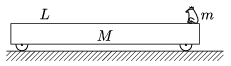(5 pont)

statistics

P. 4121. A piston, which can move without friction, closes a sample of Helium in a vertical, thermally insulated cylinder, which is closed at its bottom, and the cross-section of which is A=2 dm2. Above the piston there is a fixed stand to which an unstretched spring of spring constant D=2000 N/m is attached, whose bottom end is at a distance of d=2 dm from the piston when the volume of the gas is V0=8 dm3. The external pressure is p0=105 Pa, g=10 m/s2. The gas confined in the cylinder is heated with an electric heating element which is automatically controlled such that the piston is continuously moving with a constant speed of v0=5 cm/s.

a) Determine and plot the instantaneous power of the heating element as a function of the time, while the volume of the gas increases from V0 to V1=18 dm3.

b) Determine the heat transferred by the electric heating element to the expanding gas in the process.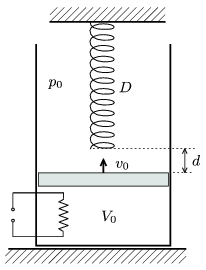(5 pont)

statistics

P. 4122. What quantity of charge and into which direction moves through the piece of conductor AB, if the switch is closed?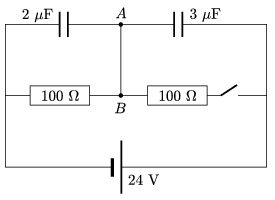(4 pont)

statistics

P. 4123. A 5 dioptre converging lens and a concave mirror of radius 40 cm are at a distance of 40 cm. The two optical devices have the same principal axis. The mirror is facing towards the lens with its reflecting side. There is a small beetle 30 cm away from the lens on the side which is opposite to the mirror.

What type of image - or images - are formed about the beetle by the optical system? What is the magnification?

(4 pont)

statistics